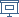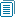FLOC 2018: FEDERATED LOGIC CONFERENCE 2018
HDRA ON SATURDAY, JULY 7TH

View: session overviewtalk overviewside by side with other conferences

09:00-10:30 Session 23D: Invited talk: Emily Riehl
Location: Blavatnik LT2
09:00
Homotopy coherent adjunctions and other structures

ABSTRACT. Naturally occurring diagrams in algebraic topology are commutative up to homotopy, but not on the nose. It was quickly realized that very little can be done with mere “homotopy commutativity." Homotopy coherent category theory arose out of a desire to catalog the higher homotopical information required to restore constructibility (or more precisely, functoriality) in such "up to homotopy" settings. In this talk we’ll focus on the syntactic categories that index homotopy coherent diagrams, which must satisfy a “freeness” or “cofibrancy” property that we axiomatize under the name “simplicial computads.” We then present as many explicit examples as time permits, including Dwyer-Kan free resolutions (which define the shape of a homotopy coherent diagram indexed by a 1-category), the homotopy coherent simplices (which are special cases), and the homotopy coherent realization of a simplicial set (a common generalization of these notions). A final example is given by the free homotopy coherent adjunction, a simplicial computad supported by a convenient graphical calculus developed in joint work with Dominic Verity.

10:00
Merge-bicategories: towards semi-strictification of higher categoriesABSTRACT. A 2-polygraph is regular if its 2-cells have non-degenerate, “interval-shaped” input and output boundaries. A merge-bicategory is a regular 2-polygraph with an algebraic composition of 2-cells, where the composable diagrams are the “disk-shaped” ones. A merge-bicategory is representable if it contains enough “divisible” 1-cells and 2-cells, satisfying certain universal properties.

I will show that representable merge-bicategories and morphisms that preserve divisible cells are equivalent to bicategories and (pseudo)functors; through a natural monoidal biclosed structure on merge-bicategories, one can also recover higher morphisms. I will use this to develop a semi-strictification argument for bicategories, combining the explicit combinatorial aspects of string-diagram based coherence proofs, with the main features of Hermida's abstract proof based on representable multicategories. All the constructions can be generalised to higher dimensions: I will sketch how this could lead to semi-strictification (excluding weak units) for higher categories, where it is still an open problem.

10:30-11:00Coffee Break
11:00-12:30 Session 26D
Location: Blavatnik LT2
11:00
The equivalence between opetopic sets and many-to-one polygraphsABSTRACT. From the polynomial approach to the definition of opetopes of Kock et al. , we derive a category \$\mathbb{O}\$ of opetopes, and show that its \$Set\$-valued presheaves, or opetopic sets, are equivalent to many-to-one polygraphs.

As an immediate corollary, we establish that opetopic sets are equivalent to multitopic sets, introduced and studied by Makkai et al. [3, 4].

11:30
Minimal models for monomial algebrasABSTRACT. Using combinatorics of chains going back to works of Anick, Green, Happel and Zacharia, we give, for any monomial algebra A, an explicit description of its minimal model. This also provides us with formulas for a canonical A-infinity-structure on the Ext-algebra of the trivial A-module.

12:00
Termination in linear (2,2)-categories with braidings and duals

ABSTRACT. This work is part of a research project aiming at developing rewriting methods to study diagrammatic algebras. In particular, we are interested in this work to diagrammatic algebras which can be seen as linear~(2,2) categories with an additional structure, for instance given by braidings, adjunctions or duals.

We present new termination heuristics for linear (3,2)-polygraphs presenting these linear (2,2)-categories based on the definition of an order similar to a monomial order, but that is not required to be total. This order is defined by counting the generators on the diagrams, and finding characteristics which are stable by contexts. We illustrate these methods by proving termination of a particular linear (3,2)-polygraph presenting a candidate 2-category for categorifying a quantum group.

12:30-14:00Lunch Break
15:00-15:30 Session 30A
Location: Blavatnik LT2
15:00
Towards a fully formalized definition of syllepsis in weak higher categories

ABSTRACT. The aim of this presentation is to illustrate the use of formal methods in order to reason in the theory of weak mega-categories. The formalism considered here is based on the type theory CaTT introduced by Finster and Mimram, extended with some metatheory on top of it, with an implementation

We first present how the system works and can be used, and then develop some ``real-world'' examples, such as the definition of the braidings in k-tuply monoidal omega-categories (following the terminology of Baez), k>= 2, with the aim of showing that we have a syllepsis in the case k<= 3. These developments have motivated metatheoretical improvements to the proof assistant, which we also present and discuss here, in order to handle and partly automate large proofs.

15:30-16:00Coffee Break
16:00-18:00 Session 31D
Location: Blavatnik LT2
16:00
Minimal Bacus FP is Turing CompleteABSTRACT. In his 1977 Turing Award address, John Backus introduced the model of functional programming called "FP". FP is a descendant of the Herbrand-Godel notion of recursive definablity and the ancestor of the programming language Haskell. One reason that FP is attractive is that it provides "an algebra of functional programs" However, Backus did not believe that basic FP was powerful enough; "FP systems have a number of limitations..... If the set of primitive functions and functional forms is weak, it may not be able to express every computable function." and he moved on to stronger systems. It turns out that, in this respect, Backus was mistaken. Here we shall show that FP can compute every partial recursive function. Indeed we shall show that FP can simulate the behavior of an arbitrary Turing machine. Our method for doing this is the following. We first observe that the equational theory of Cartesian monoids is a fragment of FP. Cartesian monoids are rather simple algebraic structures of which you know many examples. They also travel under many assumed names such as Cantor algebras, Jonsson-Tarski algebras, and Freyd-Heller monoids. This theory, together with fixed points for all Cartesian monoid polynomials, is contained in FP. Now Cartesian monoids with fixed points can be studied using rewrite techniques, learned from lambda calculus, including confluence and standarization. Turing machines can then be simulated; transitions corresponding to fixed points and computations corresponding to standard reductions to normal form.

16:30
String data structures for Chinese monoids

ABSTRACT. The structure of Chinese monoid appeared in the classification of monoids with the growth function coinciding with that of the plactic monoid. In this work, we deal with the presentations of the Chinese monoid from the rewriting theory perspective using the notion of string data structures. We define a string data structure associated to the Chinese monoid using the insertion algorithm on Chinese staircases. As a consequence, we construct a finite semi-quadratic convergent presentation of the Chinese monoid and we extend it into a finite coherent presentation of this monoid.

17:00
Generalizations of the associative operad and convergent rewrite systemsABSTRACT. The associative operad is the quotient of the magmatic operad by the operad congruence identifying the two binary trees of degree \$2\$. We introduce here a generalization of the associative operad depending on a nonnegative integer \$d\$, called \$d\$-comb associative operad, as the quotient of the magmatic operad by the operad congruence identifying the left and the right comb binary trees of degree \$d\$. We study the case \$d = 3\$ and provide an orientation of its space of relations by using rewrite systems on trees and the Buchberger algorithm for operads to obtain a convergent rewrite system.

17:30
Normal forms for planar connected string diagrams

ABSTRACT. In the graphical calculus of planar string diagrams, equality is generated by the left and right exchange moves, which swaps the heights of adjacent vertices. We show that for connected diagrams the left- and right-handed exchanges each give strongly normalizing rewrite strategies. We show that these strategies terminate in O(n^3) steps where n is the number of vertices. We also give an algorithm to directly construct the normal form, and hence determine isotopy, in O(n∙m) time, where m is the number of edges.

19:45-22:00 Workshops dinner at Balliol College

Workshops dinner at Balliol College. Drinks reception from 7.45pm, to be seated by 8:15 (pre-booking via FLoC registration system required; guests welcome).

Location: Balliol College